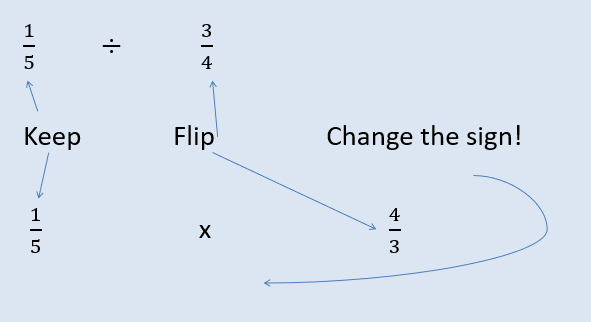# Calculations with Fractions

When we add or subtract fractions we need to make sure that the denominator is the same. The denominator tells you the size of the fraction, how many parts the whole has been split into, not the amount of fractions you have.

For example: If I have a slice of cake that is a 1/5 of the cake, I would need 5, 1/5 sized slices to make it into one whole cake.Fractions are the perfect excuse for cake!The denominator stays as a 5 and the numerators are added together!

Therefore when we have fractions with different denominators we need to find equivalent fractions to make sure the denominators are the same.

Eg: 2/7 + 1/6 =

Multiply the denominators by each other to find a common multiple,

7x6 = 42 so a denominator of 42 will create equivalent fractions.

2/7 = 12/42. 1/6 = 7/42.

Now we have two fractions that we can add together: 12/42 + 7/42 = 19/42. If necessary we would then cancel down the fractions

## Multiplying Fractions

To multiply simply multiply the numerator by the numerator and the denominator by the denominator!

Eg. 2/7 x 1/5 = 2x1 / 7x5 = 2/35.

## Dividing Fractions

To divide fractions, go to KFC= Keep, Flip, Change:= 4/15